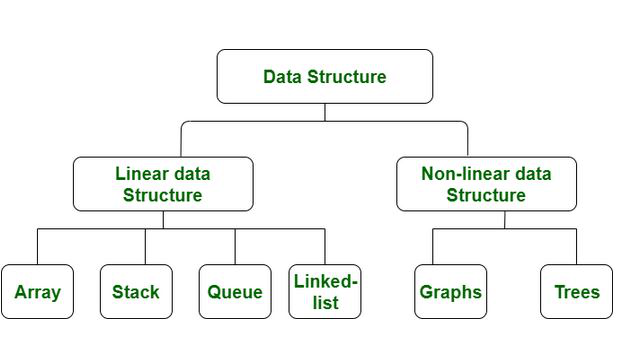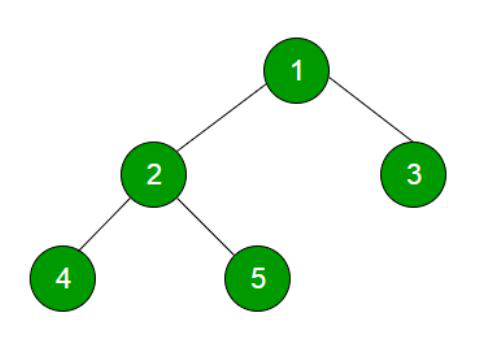GeeksforGeeks App
Open AppBrowser
Continue

# Implementing Inorder, Preorder, Postorder Using Stack in Java

Data structures of two types of Linear Data Structure and the second is Non-linear Data Structure the main difference between these Data structures is the way of transverse the elements of these data structures. Linear Data Structure Transverse all elements in a single run whereas Non-Linear Data structure Transverse all elements in Hierarchy way.In this article, we will implement different types of Depth First Traversals in the Binary Tree of Non-Linear Data structure using the stack data structure.

Input Binary Tree:Depth First Traversals:

1. Inorder (Left, Root, Right) : 4 2 5 1 3
2. Preorder (Root, Left, Right) : 1 2 4 5 3
3. Postorder (Left, Right, Root) : 4 5 2 3 1

### A. Inorder Traversal

1. Create an empty stack S.

2. Initialize the current node as root.

3. Push the current node to S and set current = current->left until the current is NULL.

4. If the current is NULL and the stack is not empty then

• Pop the top item from the stack.
• Print the popped item, set current = popped_item->right
• Go to step 3.

5. If the current is NULL and the stack is empty then we are done.

Below is the implementation of the above approach:

## Java

 `// Non-Recursive Java program for inorder traversal``import` `java.util.Stack;` `// Class containing left and right child of``// current node and key value``class` `Node {``    ``int` `data;``    ``Node left, right;` `    ``public` `Node(``int` `item)``    ``{``        ``data = item;``        ``left = right = ``null``;``    ``}``}` `// Class to print the inorder traversal``class` `BinaryTree {``    ``Node root;``    ``void` `inorder()``    ``{``        ``if` `(root == ``null``)``            ``return``;` `        ``Stack s = ``new` `Stack();``        ``Node curr = root;` `        ``// traverse the tree``        ``while` `(curr != ``null` `|| s.size() > ``0``) {` `            ``// Reach the left most``            ``// Node of the current Node``            ``while` `(curr != ``null``) {``                ``// place pointer to a tree node on``                ``// the stack before traversing``                ``// the node's left subtree``                ``s.push(curr);``                ``curr = curr.left;``            ``}` `            ``// Current must be NULL at this point``            ``curr = s.pop();` `            ``System.out.print(curr.data + ``" "``);` `            ``// we have visited the node and its``            ``// left subtree. Now, it's right``            ``// subtree's turn``            ``curr = curr.right;``        ``}``    ``}` `    ``public` `static` `void` `main(String args[])``    ``{` `        ``// Creating a binary tree and``        ``// entering the nodes``        ``BinaryTree tree = ``new` `BinaryTree();``        ``tree.root = ``new` `Node(``1``);``        ``tree.root.left = ``new` `Node(``2``);``        ``tree.root.right = ``new` `Node(``3``);``        ``tree.root.left.left = ``new` `Node(``4``);``        ``tree.root.left.right = ``new` `Node(``5``);``        ``tree.inorder();``    ``}``}`

Output

`4 2 5 1 3`
• TimeComplexity: O(n)
• SpaceComplexity: O(n)

### B. Preorder Traversal

There are two approaches for Preorder Traversal of Binary Tree using the Stack data structure:

Approach 1: This approach is totally the same which discussed in the above Traversal.

1. Create an empty stack S.
2. Initialize the current node as root.
3. Push the current node to S and set current = current->left print the peek element in the stack until the current is NULL.
4. If current is NULL and stack is not empty then
a) Pop the top item from stack.
b) set current = popped_item->right.
c) Go to step 3.
5. If the current is NULL and the stack is empty then we are done.

Below is the implementation of the above approach:

## Java

 `// Java Program for Pre order Traversal``import` `java.util.*;``import` `java.io.*;``class` `Node {``    ``int` `data;``    ``Node left, right;` `    ``public` `Node(``int` `item)``    ``{``        ``data = item;``        ``left = right = ``null``;``    ``}``}` `// Class to print the preorder traversal``class` `BinaryTree {``    ``Node root;``    ``void` `preorder()``    ``{``        ``if` `(root == ``null``)``            ``return``;` `        ``Stack s = ``new` `Stack();``        ``Node curr = root;` `        ``// traverse the tree``        ``while` `(curr != ``null` `|| s.size() > ``0``) {` `            ``// Reach the left most``            ``// Node of the curr Node``            ``while` `(curr != ``null``) {``                ``// place pointer to a tree node on``                ``// the stack before traversing``                ``// the node's left subtree``                ``s.push(curr);``                ``// print the peak element``                ``System.out.print(s.peek().data + ``" "``);` `                ``curr = curr.left;``            ``}` `            ``// Current must be NULL at this point``            ``curr = s.pop();` `            ``// we have visited the node and its``            ``// left subtree. Now, it's right``            ``// subtree's turn``            ``curr = curr.right;``        ``}``    ``}` `    ``public` `static` `void` `main(String args[])``    ``{` `        ``// creating a binary tree and``        ``// entering the nodes``        ``BinaryTree tree = ``new` `BinaryTree();``        ``tree.root = ``new` `Node(``1``);``        ``tree.root.left = ``new` `Node(``2``);``        ``tree.root.right = ``new` `Node(``3``);``        ``tree.root.left.left = ``new` `Node(``4``);``        ``tree.root.left.right = ``new` `Node(``5``);``        ``tree.preorder();``    ``}``}`

Output

`1 2 4 5 3`
• Time Complexity: O(n)
• Space Complexity: O(n)

Approach 2: In Preorder Traversal, First print the root element first then the left subtree, and then the right subtree. We know that Stack data structure follows LIFO(Last in First Out), So we take the advantage of this feature of this stack we first push the right part of the current tree and after the left part of the current tree, and in every iteration, we pop the peak element from stack and print and then again push right part of the pop element and left part of the pop element till the size of the stack is not equal to 1 because we have already printed the first element.

Algorithm:

• Create an empty stack S.
• Print the root element.
• Push the right subtree to the stack.
• Push the left subtree to the stack.
• If the stack size is not 1 then
• Pop the top item from the stack.
• print the element
• Push right Subtree of pop element to the stack.
• Push left  Subtree of pop element to the stack.
• If the Size of the stack is 1 return to the main method

Below is the implementation of the above approach:

## Java

 `// Non-Recursive Java Program for preorder traversal``import` `java.util.Stack;``// Class containing left and right child of``// current node and key value``class` `Node {``    ``int` `data;``    ``Node left, right;` `    ``public` `Node(``int` `item)``    ``{``        ``data = item;``        ``left = right = ``null``;``    ``}``}` `// Class to print the preorder traversal``class` `BinaryTree {``    ``Node root;``    ``void` `preorder()``    ``{``        ``if` `(root == ``null``) {``            ``return``;``        ``}``        ``Stack S = ``new` `Stack<>();` `        ``// Push root element in the stack``        ``S.add(root);` `        ``// print the root element``        ``System.out.print(root.data + ``" "``);` `        ``// Push right subtree to the stack``        ``if` `(root.right != ``null``) {``            ``S.add(root.right);``        ``}``        ``// Push left subtree to the stack``        ``if` `(root.left != ``null``) {``            ``S.add(root.left);``        ``}``        ``// Iterate till Size of the Stack not equal to 1``        ``while` `(S.size() != ``1``) {``            ``// Peek element of the stack``            ``Node temp = S.peek();``            ``// pop the element from the stack``            ``S.pop();` `            ``if` `(temp != ``null``) {``                ``// print the pop element``                ``System.out.print(temp.data + ``" "``);` `                ``// Push right subtree of the pop element``                ``if` `(temp.right != ``null``) {``                    ``S.add(temp.right);``                ``}``                ``// Push left subtree of the pop element``                ``if` `(temp.left != ``null``) {``                    ``S.add(temp.left);``                ``}``            ``}``        ``}``    ``}` `    ``public` `static` `void` `main(String args[])``    ``{` `        ``// creating a binary tree and``        ``// entering the nodes``        ``BinaryTree tree = ``new` `BinaryTree();``        ``tree.root = ``new` `Node(``1``);``        ``tree.root.left = ``new` `Node(``2``);``        ``tree.root.right = ``new` `Node(``3``);``        ``tree.root.left.left = ``new` `Node(``4``);``        ``tree.root.left.right = ``new` `Node(``5``);``        ``tree.preorder();``    ``}``}`

Output

`1 2 4 5 3`
• TimeComplexity: O(n)
• SpaceComplexity: O(n)

### C. PostOrder Traversal

1. Create an empty stack

2. Do the following while the root is not NULL

• Push root’s right child and then the root to stack.
• Set root as root’s left child.

3. Pop an item from the stack and set it as root.

• If the popped item has a right child and the right child is at top of the stack, then remove the right child from the stack, push the root back, and set the root as root’s right child.
• Else print root’s data and set root as NULL.

4. Repeat steps 2 and 3 while the stack is not empty.

Below is the implementation of the above approach:

## Java

 `// Java program for iterative postorder``// traversal using stack` `import` `java.util.ArrayList;``import` `java.util.Stack;` `// A binary tree node``class` `Node {``    ``int` `data;``    ``Node left, right;` `    ``Node(``int` `item)``    ``{``        ``data = item;``        ``left = right;``    ``}``}` `class` `BinaryTree {``    ``Node root;` `    ``// An iterative function to do postorder traversal``    ``// of a given binary tree``    ``void` `postOrder(Node node)``    ``{``        ``Stack S = ``new` `Stack();` `        ``// Check for empty tree``        ``if` `(node == ``null``)``            ``return``;``        ``S.push(node);``        ``Node prev = ``null``;``        ``while` `(!S.isEmpty()) {``            ``Node current = S.peek();` `            ``// go down the tree in search of a leaf an if so``            ``// process it and pop stack otherwise move down``            ``if` `(prev == ``null` `|| prev.left == current``                ``|| prev.right == current) {``                ``if` `(current.left != ``null``)``                    ``S.push(current.left);``                ``else` `if` `(current.right != ``null``)``                    ``S.push(current.right);``                ``else` `{``                    ``S.pop();``                    ``System.out.print(current.data + ``" "``);``                ``}` `                ``// go up the tree from left node, if the``                ``// child is right push it onto stack``                ``// otherwise process parent and pop``            ``}``            ``else` `if` `(current.left == prev) {``                ``if` `(current.right != ``null``)``                    ``S.push(current.right);``                ``else` `{``                    ``S.pop();``                    ``System.out.print(current.data + ``" "``);``                ``}` `                ``// go up the tree from right node and after``                ``// coming back from right node process parent``                ``// and pop stack``            ``}``            ``else` `if` `(current.right == prev) {``                ``S.pop();``                ``System.out.print(current.data + ``" "``);``            ``}` `            ``prev = current;``        ``}``    ``}` `    ``// Driver program to test above functions``    ``public` `static` `void` `main(String args[])``    ``{``        ``BinaryTree tree = ``new` `BinaryTree();` `        ``// Let us create trees shown in above diagram``        ``tree.root = ``new` `Node(``1``);``        ``tree.root.left = ``new` `Node(``2``);``        ``tree.root.right = ``new` `Node(``3``);``        ``tree.root.left.left = ``new` `Node(``4``);``        ``tree.root.left.right = ``new` `Node(``5``);` `        ``tree.postOrder(tree.root);``    ``}``}`

Output

`4 5 2 3 1`
• Time Complexity: O(n)
• Space Complexity: O(n)

My Personal Notes arrow_drop_up